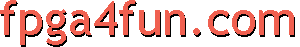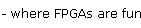## Pong Game

FPGAs can become video generators easily.The pong game consists of a ball bouncing on a screen. A paddle (controlled from a mouse here) enables the user to make the ball bounce back up.

We use a Pluto FPGA board, although any other FPGA development board would work.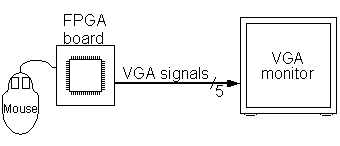#### Driving a VGA monitor

A VGA monitor requires 5 signals to display a picture:
• R, G and B (red, green and blue signals).
• HS and VS (horizontal and vertical synchronization).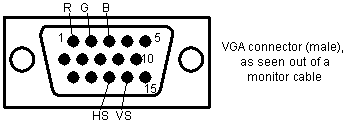The R, G and B are analog signals, while HS and VS are digital signals.

#### Creating a VGA video signal from FPGA pins

Here's how to drive the VGA connector: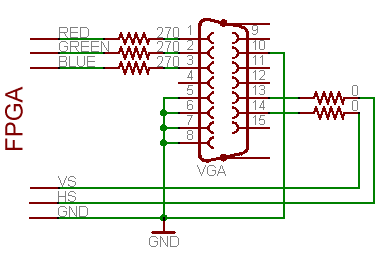• Pins 13 and 14 of the VGA connector (HS and VS) are digital signals, so can be driven directly from two FPGA pins (or through low value resistors, like 100Ω).
• Pins 1, 2 and 3 (R, G and B) are 75Ω analog signals with nominal values of 0.7V. With 3.3V FPGA outputs, use three 270Ω series resistors. The resistors form voltage dividers with the 75Ω resistors in the monitor inputs so that 3.3V become 3.3*75/(270+75)=0.72V, pretty close to 0.7V. Driving these RGB pins with different combinations of 0's and 1's gives us up to 8 colors.
• Ground pins are pins 5, 6, 7, 8 and 10.

Here's a view of the female VGA connector connected to Pluto on a breadboard.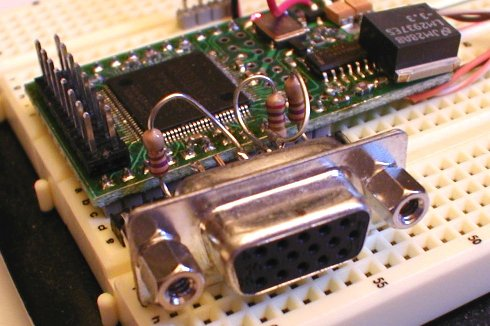Back view of the female VGA connector to 12-pins header assembly. The 12-pins header makes it easy to connect to a breadboard. The three 270Ω series resistors are clearly visible. We could also have used an adapter board.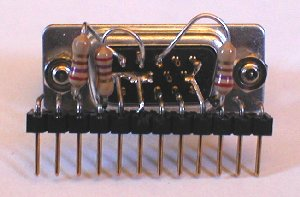#### Frequency generator

A monitor always displays a picture line-by-line, from top-to-bottom. Each line is drawn from left-to-right.

That's hard-coded, you cannot change that.

But you specify when the drawing starts by sending short pulses on HS and VS at fixed intervals. HS makes a new line to start drawing; while VS tells that the bottom has been reached (makes the monitor go back up to the top line).

For the standard 640x480 VGA video signal, the frequencies of the pulses should be:

Vertical Freq (VS)Horizontal Freq (HS)
60 Hz (=60 pulses per second)31.5 kHz (=31500 pulses per second)

To create a standard video signal, there is more details to take care of, like the duration of the pulses and the relationship between HS and VS. Get an idea on this page.

#### Our first video generator

Nowadays, VGA monitors are multisync, so can accommodate non-standard frequencies - no need to generate exactly 60Hz and 31.5KHz anymore (but if you are using an old (non-multisync) VGA monitor, you'll need to generate the exact frequencies).

 reg [9:0] CounterX; reg [8:0] CounterY; wire CounterXmaxed = (CounterX==767); always @(posedge clk) if(CounterXmaxed)   CounterX <= 0; else   CounterX <= CounterX + 1; always @(posedge clk) if(CounterXmaxed)     CounterY <= CounterY + 1;

CounterX counts 768 values (from 0 to 767) and CounterY counts 512 values (0 to 511).

Now, CounterX is used to generate HS, and CounterY to generate VS. Using a 25MHz clock, we get 32.5KHz for HS and 63.5Hz for VS. The pulses need to be active long enough for the monitor to detect them. Let's use a 16 clocks pulse (0.64µs) for HS and a full horizontal line length pulse for VS (768 clocks or 30µs). That's shorter than what the VGA spec calls for but works fine anyway.

We generate the HS and VS pulses from D flip-flop (to get glitch free outputs).

 reg vga_HS, vga_VS; always @(posedge clk) begin   vga_HS <= (CounterX[9:4]==0);   // active for 16 clocks   vga_VS <= (CounterY==0);   // active for 768 clocks end

The VGA outputs need to be negative, so we invert the signals.

 assign vga_h_sync = ~vga_HS; assign vga_v_sync = ~vga_VS;

Finally we can drive the R, G and B signals. As a first cut, we can use some bits of the X and Y counters to get nice square color patterns...

 assign R = CounterY | (CounterX==256); assign G = (CounterX ^ CounterX) | (CounterX==256); assign B = CounterX | (CounterX==256);

... and we get a picture on the VGA monitor!

#### Drawing a useful picture

The sync generator is best rewritten to be used as an HDL module where we generate R, G and B outside. Also the X and Y counters are more useful if they start counting from the drawing area.
The new file can be found here.

Now we can use it to draw a border around the screen.

 module pong(clk, vga_h_sync, vga_v_sync, vga_R, vga_G, vga_B); input clk; output vga_h_sync, vga_v_sync, vga_R, vga_G, vga_B; wire inDisplayArea; wire [9:0] CounterX; wire [8:0] CounterY; hvsync_generator syncgen(.clk(clk), .vga_h_sync(vga_h_sync), .vga_v_sync(vga_v_sync),                             .inDisplayArea(inDisplayArea), .CounterX(CounterX), .CounterY(CounterY)); // Draw a border around the screen wire border = (CounterX[9:3]==0) || (CounterX[9:3]==79) || (CounterY[8:3]==0) || (CounterY[8:3]==59); wire R = border; wire G = border; wire B = border; reg vga_R, vga_G, vga_B; always @(posedge clk) begin   vga_R <= R & inDisplayArea;   vga_G <= G & inDisplayArea;   vga_B <= B & inDisplayArea; end endmodule

Let's use a mouse to move the paddle left and right on the screen.

The quadrature decoder page shows the secret. The code is as follow:

 wire border = (CounterX[9:3]==0) || (CounterX[9:3]==79) || (CounterY[8:3]==0) || (CounterY[8:3]==59); wire paddle = (CounterX>=PaddlePosition+8) && (CounterX<=PaddlePosition+120) && (CounterY[8:4]==27); wire R = border | (CounterX ^ CounterY) | paddle; wire G = border | paddle; wire B = border | paddle;

#### Drawing the ball

The ball needs to move around the screen, and bounce back when it touches an object (border or paddle).

First we display the ball. It is a square 16x16 pixels. We activate the drawing of the ball when CounterX and CounterY reach its coordinates.

 reg [9:0] ballX; reg [8:0] ballY; reg ball_inX, ball_inY; always @(posedge clk) if(ball_inX==0) ball_inX <= (CounterX==ballX) & ball_inY; else ball_inX <= !(CounterX==ballX+16); always @(posedge clk) if(ball_inY==0) ball_inY <= (CounterY==ballY); else ball_inY <= !(CounterY==ballY+16); wire ball = ball_inX & ball_inY;

Now for the collisions. That's the difficult part of this project.

We could check the coordinate of the ball against each object on the screen and determine if there is a collision. But that would become quickly a nightmare as the number of objects increases.

Instead we define 4 "hot-spots" pixels, one in the middle of each side of the ball. If an object (border or paddle) redraws itself at the same time that the ball draws one of its "hot-spot", we know that there is collision on that side of the ball.

 wire border = (CounterX[9:3]==0) || (CounterX[9:3]==79) || (CounterY[8:3]==0) || (CounterY[8:3]==59); wire paddle = (CounterX>=PaddlePosition+8) && (CounterX<=PaddlePosition+120) && (CounterY[8:4]==27); wire BouncingObject = border | paddle; // active if the border or paddle is redrawing itself reg CollisionX1, CollisionX2, CollisionY1, CollisionY2; always @(posedge clk) if(BouncingObject & (CounterX==ballX ) & (CounterY==ballY+ 8)) CollisionX1<=1; always @(posedge clk) if(BouncingObject & (CounterX==ballX+16) & (CounterY==ballY+ 8)) CollisionX2<=1; always @(posedge clk) if(BouncingObject & (CounterX==ballX+ 8) & (CounterY==ballY )) CollisionY1<=1; always @(posedge clk) if(BouncingObject & (CounterX==ballX+ 8) & (CounterY==ballY+16)) CollisionY2<=1;

(I simplified a little the above code by never resetting the collision flops, the complete code is available below).

Now we update the ball position, but only once for every video frame.

 reg UpdateBallPosition;       // active only once for every video frame always @(posedge clk) UpdateBallPosition <= (CounterY==500) & (CounterX==0); reg ball_dirX, ball_dirY; always @(posedge clk) if(UpdateBallPosition) begin   if(~(CollisionX1 & CollisionX2))        // if collision on both X-sides, don't move in the X direction   begin     ballX <= ballX + (ball_dirX ? -1 : 1);     if(CollisionX2) ball_dirX <= 1; else if(CollisionX1) ball_dirX <= 0;   end   if(~(CollisionY1 & CollisionY2))        // if collision on both Y-sides, don't move in the Y direction   begin     ballY <= ballY + (ball_dirY ? -1 : 1);     if(CollisionY2) ball_dirY <= 1; else if(CollisionY1) ball_dirY <= 0;   end end

And finally we can draw all that together.

 wire R = BouncingObject | ball | (CounterX ^ CounterY); wire G = BouncingObject | ball; wire B = BouncingObject | ball; reg vga_R, vga_G, vga_B; always @(posedge clk) begin   vga_R <= R & inDisplayArea;   vga_G <= G & inDisplayArea;   vga_B <= B & inDisplayArea; end

Whoa, wasn't so difficult after all.
The complete file is pong.zip, and works with hvsync_generator.zip

It is also possible to use HDMI to run the pong game.